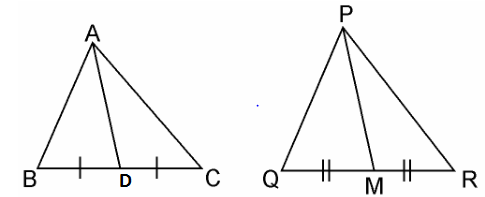Guru

# If AD and PM are medians of triangles ABC and PQR, respectively where ΔABC ~ ΔPQR prove that AB/PQ = AD/PM. Q.16

• 0

The best way to solve the question of Triangles of exercise 6.3 of class 10th math, please suggest me the best way to solve this problem If AD and PM are medians of triangles ABC and PQR, respectively where ΔABC ~ ΔPQR prove that AB/PQ = AD/PM.

Share

1. Given, ΔABC ~ ΔPQRWe know that the corresponding sides of similar triangles are in proportion.

∴AB/PQ = AC/PR = BC/QR……………………………(i)

Also, ∠A = ∠P, ∠B = ∠Q, ∠C = ∠R ………….…..(ii)

Since AD and PM are medians, they will divide their opposite sides.

∴ BD = BC/2 and QM = QR/2 ……………..………….(iii)

From equations (i) and (iii), we get

AB/PQ = BD/QM ……………………….(iv)

In ΔABD and ΔPQM,

From equation (ii), we have

∠B = ∠Q

From equation (iv), we have,

AB/PQ = BD/QM

∴ ΔABD ~ ΔPQM (SAS similarity criterion)